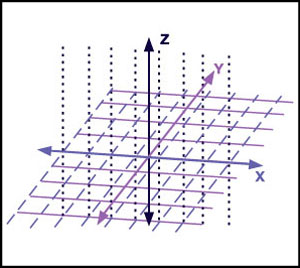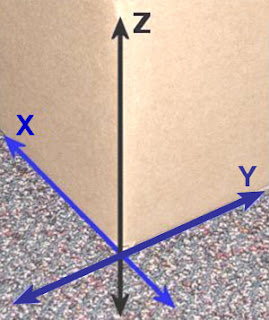### Positive and Negative DirectionsFigure 1. Each axis has a positive and negative direction.Figure 2. The positive direction of the Y-axis points away, and the positive direction of the Z-axis points upward.
Each axis line contains a range of numbers. The origin, or the center of the axis line, is always zero. Numbers are then counted as they move away from the center on each side of the axis. One direction is positive (+), and the other is negative (-).

Measurements taken to the right side of the origin along the X-axis line are generally considered to be positive, or +x, while measurements to the left of the origin along the X-axis are negative, or –x, as shown in Figure 1. The positive and negative directions work the same way for the Y- and Z-axes as well.

Figure 2 shows the box corner. The positive direction of the Y-axis points away and the positive direction of the Z-axis points upward.